# Heat Transfer Through Fins Notes | Study Heat Transfer - Mechanical Engineering

## Mechanical Engineering: Heat Transfer Through Fins Notes | Study Heat Transfer - Mechanical Engineering

The document Heat Transfer Through Fins Notes | Study Heat Transfer - Mechanical Engineering is a part of the Mechanical Engineering Course Heat Transfer.
All you need of Mechanical Engineering at this link: Mechanical Engineering

Heat Transfer from Extended Surface (Fin)

• A fin is a surface that extends from an object to increase the rate of heat transfer to or from the environment by increase convection.
• Adding a fin to an object increases the surface area and can sometimes be an economical solution to heat transfer problems.
• Finned surfaces are commonly used in practice to enhance heat transfer. In the analysis of the fins, we consider steady operation with no heat generation in the fin.
• We also assume that the convection heat transfer coefficient h to be constant and uniform over the entire surface of the fin.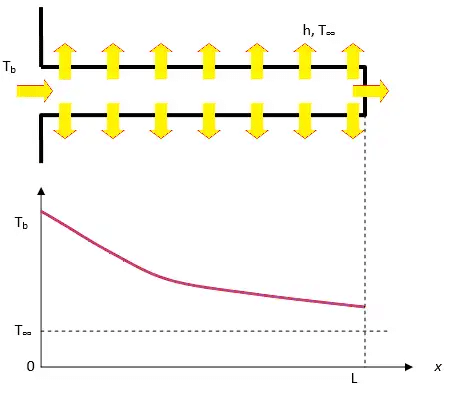• The rate of heat transfer from a solid surface to atmosphere is given by Q = hA ∆ T where, h and ∆T are not controllable.
• So, to increase the value of Q surface area should be increased. The extended surface which increases the rate of heat transfer is known as fin.

Generalized Equation for Fin Rectangular Fin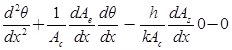Where Ac and As are cross-sectional and surface area:
And θ(x) = t(x)–ta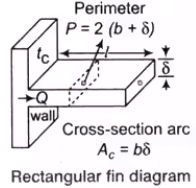Heat balance equation if Ac constant and As ∞ P(x) linear:• General equation of 2nd order: θ = c1emx + c2e–mx
• Heat dissipation can take place on the basis of three cases.

Case 1: Heat Dissipation from an Infinitely Long Fin (l → ∞):

• In such a case, the temperature at the end of Fin approaches to surrounding fluid temperature ta as shown in figure. The boundary conditions are given below
• At x = 0, t = t0 : θ = t0 – ta = θ0
• At x = L→ ∞: t = ta, θ = 0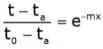θ = θ0 e–mx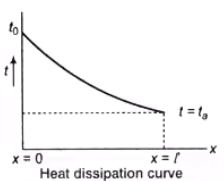• Heat transfer by conduction at base: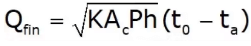Case 2: Heat Dissipation from a Fin Insulated at the End Tip:

• Practically, the heat loss from the long and thin film tip is negligible, thus the end of the tip can be, considered as insulated.
• At x = 0, t = t0 and θ = t0 – ta = θ0
at x = l , Q = 0 i.e, dθ/dx = 0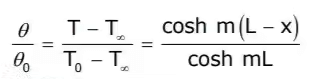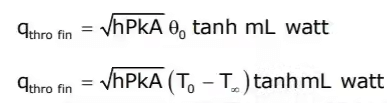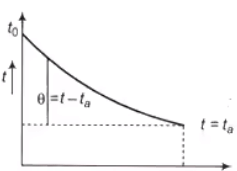Case 3: Heat Dissipation from a Fin loosing Heat at the End Tip:

• The boundary conditions are given below.
• At x = 0, t = t0 and θ = θ0
• At x = l
Qconduction = Qconvection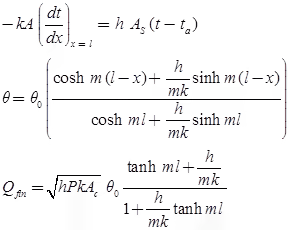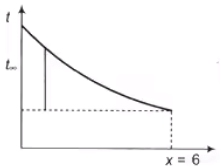Fin Efficiency

Fin efficiency is given by: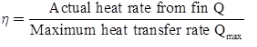• If l → ∞ (infinite length of fin):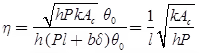• If fin is with insulated tip: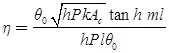• If finite length of fin: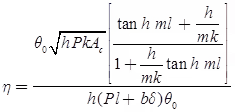Note: The following must be noted for a proper fin selection:

• The longer the fin, the larger the heat transfer area and thus the higher the rate of heat transfer from the fin.
• The larger the fin, the bigger the mass, the higher the price, and larger the fluid friction.
• The fin efficiency decreases with increasing fin length because of the decrease in fin temperature with length.

Fin Effectiveness

• The performance of fins is judged on the basis of the enhancement in heat transfer relative to the no‐fin case, and expressed in terms of the fin effectiveness:• For a sufficiently long fin of uniform cross‐section Ac, the temperature at the tip of the fin will approach the environment temperature, T. By writing energy balance and solving the differential equation, one finds: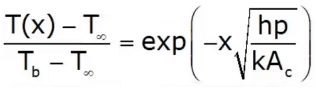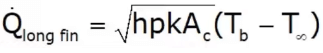where Ac is the cross‐sectional area, x is the distance from the base, and p is perimeter.  The effectiveness becomes: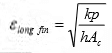To increase fins effectiveness, one can conclude:

• The thermal conductivity of the fin material must be as high as possible
• The ratio of perimeter to the cross‐sectional area p/Ac should be as high as possible
• The use of fin is most effective in applications that involve low convection heat transfer coefficient, i.e. natural convection.
The document Heat Transfer Through Fins Notes | Study Heat Transfer - Mechanical Engineering is a part of the Mechanical Engineering Course Heat Transfer.
All you need of Mechanical Engineering at this link: Mechanical EngineeringUse Code STAYHOME200 and get INR 200 additional OFF

## Heat Transfer

58 videos|70 docs|85 tests

Track your progress, build streaks, highlight & save important lessons and more!

,

,

,

,

,

,

,

,

,

,

,

,

,

,

,

,

,

,

,

,

,

;